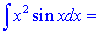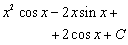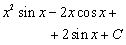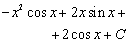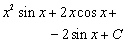Math Calculators, Lessons and Formulas

It is time to solve your math problem

mathportal.org
 « Integration by Substitution
Integration Techniques: (lesson 2 of 4)

## Integration by Parts

Theorem:

The formula for the method of integration by parts is given by: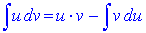Four steps to use this formula:

Step 1: Identify u and dv. Priorities for Choosing u are: 1. u = lnx 2. u = xn 3. u = eax

Step 2: Compute du and v

Step 3: Use the formula for the integration by parts

Example 1: Evaluate the following integral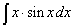Solution:

Step 1: In this example we choose u = x and dv will be everything else that remains.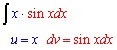Step 2: Compute du and v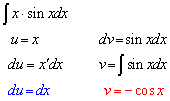Step 3: Use the formula.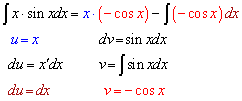Therefore: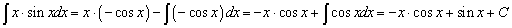Example 2: Evaluate the following integral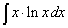Solution:

Step 1: In this example we choose u = ln x (first priority) and dv = x dx.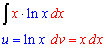Step 2: Compute du and v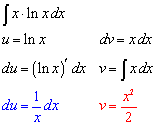Step 3: Use the formula.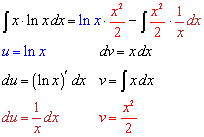The solution is: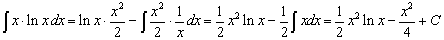Exercise 1: Evaluate the following integrals

Level 1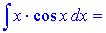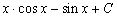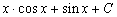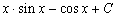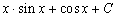Level 2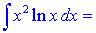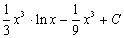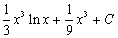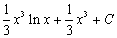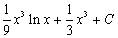### Integration by parts twice

Example 3: Evaluate the following integral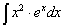Solution:

Let: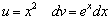So that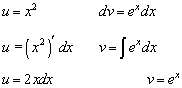Therefore: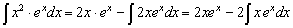We need to perform integration by parts again: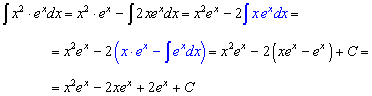Try yourself